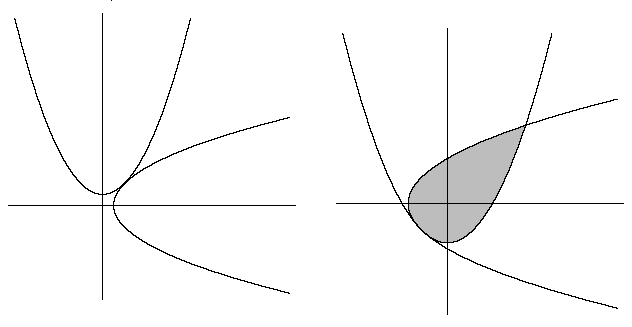# The Teardrop

Calculus Level 4

Let $c$ be a negative constant. When the two parabolas $y=x^2+c$ and $x=y^2+c$ are tangent, the area of the region of overlap can be expressed as $\frac {A}B$, where $A$ and $B$ are coprime positive integers. Find $A + B$.×

Problem Loading...

Note Loading...

Set Loading...